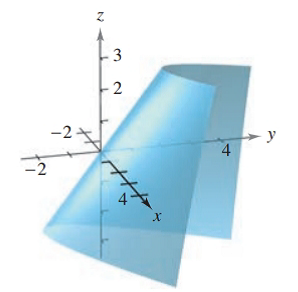Chapter 7.5, Problem 16E### Calculus: An Applied Approach (Min...

10th Edition
Ron Larson
ISBN: 9781305860919

#### Solutions

Chapter
Section### Calculus: An Applied Approach (Min...

10th Edition
Ron Larson
ISBN: 9781305860919
Textbook Problem
1 views

# Applying the Second-Partials Test In Exercises 1-18, find the relative extrema and saddle points of the function. See Examples f ( x , y ) = x + y + 2 x y − x 2 − y 2To determine

To calculate: The relative extrema and saddle points of the function f(x,y)=x+y+2xyx2y2

Explanation

Given Information:

The equation of the function is given as f(x,y)=x+y+2xyx2y2.

The provided figure is,

Formula used:

To check the relative extrema and saddle points

d=fxx(a,b)fyy(a,b)[fxy(a,b)]2

Following steps to be followed:

Step 1: If d>0 and fxx(a,b)>0 then the function has a relative minimum at (a,b).

Step 2: If d>0 and fxx(a,b)<0 then the function has a relative minimum at (a,b).

Step 3: If d<0 then (a,b,f(a,b)) is a saddle point.

Step 4: The test gains no information when d=0.

Calculation:

Consider the given equation and differentiate with respect to x and y respectively

f(x,y)=x+y+2xyx2y2fx(x,y)=1+2y2xfy(x,y)=1+2x2y

Now, fx(x,y) and fy(x,y) are defined for all points in the xy-plane

### Still sussing out bartleby?

Check out a sample textbook solution.

See a sample solution

#### The Solution to Your Study Problems

Bartleby provides explanations to thousands of textbook problems written by our experts, many with advanced degrees!

Get Started

#### In Exercises 2340, find the indicated limit. 25. limx3x

Applied Calculus for the Managerial, Life, and Social Sciences: A Brief Approach

#### True or False: If f′(c) = 0 and f″(c) < 0, then c is a local minimum.

Study Guide for Stewart's Single Variable Calculus: Early Transcendentals, 8th

#### The unit vector in the direction of is:

Study Guide for Stewart's Multivariable Calculus, 8th